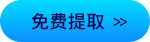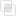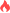python进程之间如何通信2021-03-09 16:10:14205浏览 · 0收藏 · 0评论1、思路

Process之间肯定是需要通信的，操作系统提供了很多机制来实现进程间的通信。Python的multiprocessing模块包装了底层的机制，提供了Queue、Pipes等多种方式来交换数据。

2、实例

```from multiprocessing import Process, Queue
import os, time, random

# 写数据进程执行的代码:
def write(q):
print('Process to write: %s' % os.getpid())
for value in ['A', 'B', 'C']:
print('Put %s to queue...' % value)
q.put(value)
time.sleep(random.random())

# 读数据进程执行的代码:
def read(q):
print('Process to read: %s' % os.getpid())
while True:
value = q.get(True)
print('Get %s from queue.' % value)

if __name__=='__main__':
# 父进程创建Queue，并传给各个子进程：
q = Queue()
pw = Process(target=write, args=(q,))
pr = Process(target=read, args=(q,))
# 启动子进程pw，写入:
pw.start()
# 启动子进程pr，读取:
pr.start()
# 等待pw结束:
pw.join()
# pr进程里是死循环，无法等待其结束，只能强行终止:
pr.terminate()```GIL在python的获取和释放python多进程取代多线程的探究python协程的作用python中jsonrpc的实现python分数如何使用879

python tempfile创建文件382

python finally语句如何使用206